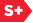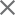### Proceedings Paper

General Hilbert space framework for the discretization of continuous signal processing operators
Author(s): Michael A. Unser
Format Member Price Non-Member Price
PDF \$17.00 \$21.00

Paper Abstract

We present a unifying framework for the design of discrete algorithms that implement continuous signal processing operators. The underlying continuous-time signals are represented as linear combinations of the integer-shifts of a generating function pi with (i equals 1,2) (continuous/discrete representation). The corresponding input and output functions spaces are V(pi) and V(p2), respectively. The principle of the method is as follows: we start by interpolating the discrete input signal with a function s1 (epsilon) V(p1). We then apply a linear operator T to this function and compute the minimum error approximation of the result in the output space V(p2). The corresponding algorithm can be expressed in terms of digital filters and a matrix multiplication. In this context, we emphasize the advantages of B-splines; and show how a judicious use of these basis functions can result in fast implementations of various types of operators. We present design examples of differential operators involving very short FIR filters. We also describe an efficient procedure for the geometric affine transformation of signals. The present formulation is general enough to include most earlier continuous/discrete signal processing techniques (e.g., standard bandlimited approach, spline or wavelet-based) as special cases.

Paper Details

Date Published: 1 September 1995
PDF: 11 pages
Proc. SPIE 2569, Wavelet Applications in Signal and Image Processing III, (1 September 1995); doi: 10.1117/12.217597
Show Author Affiliations
Michael A. Unser, National Institutes of Health (United States)

Published in SPIE Proceedings Vol. 2569:
Wavelet Applications in Signal and Image Processing III
Andrew F. Laine; Michael A. Unser, Editor(s)